Скачать презентацию macro CHAPTER FOUR Money and Inflation macroeconomics fifth

7fd40236893c13c4698c2e27ebf0f06e.ppt

• Количество слайдов: 71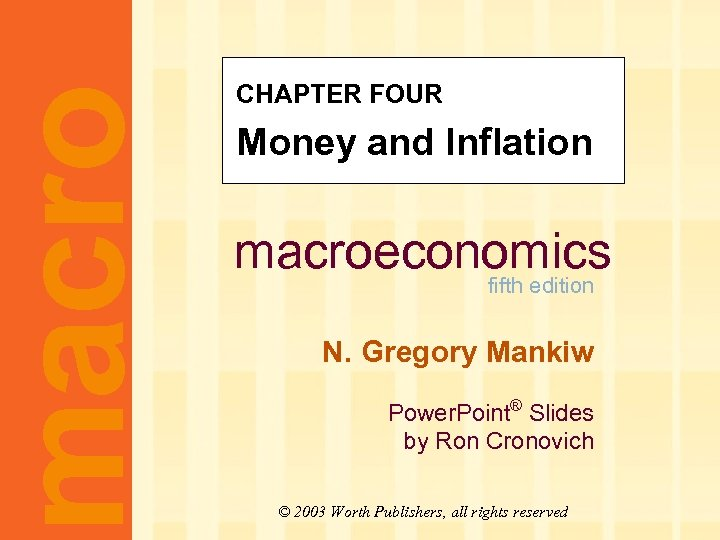macro CHAPTER FOUR Money and Inflation macroeconomics fifth edition N. Gregory Mankiw Power. Point® Slides by Ron Cronovich © 2003 Worth Publishers, all rights reserved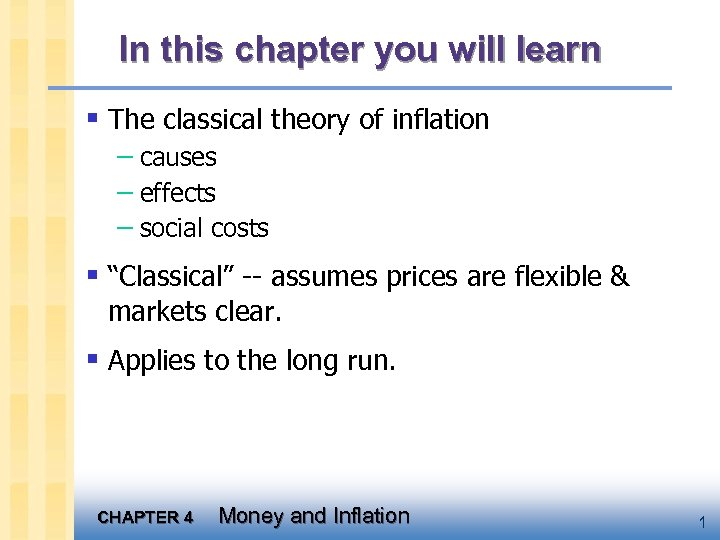In this chapter you will learn § The classical theory of inflation – causes – effects – social costs § “Classical” -- assumes prices are flexible & markets clear. § Applies to the long run. CHAPTER 4 Money and Inflation 1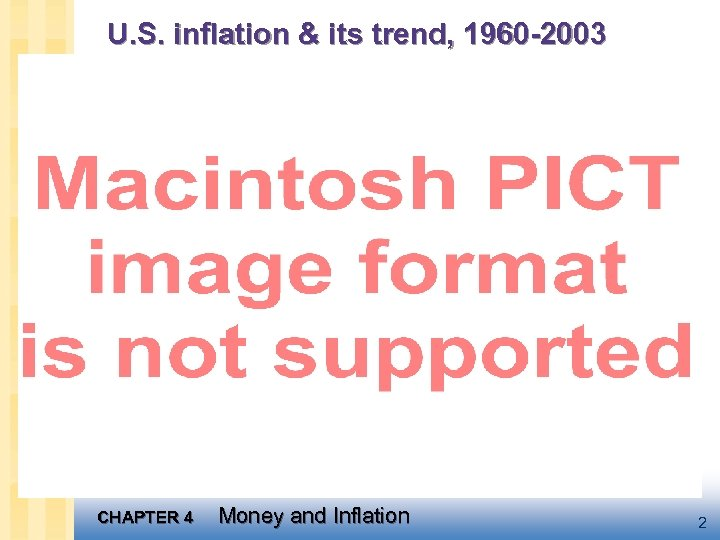U. S. inflation & its trend, 1960 -2003 CHAPTER 4 Money and Inflation 2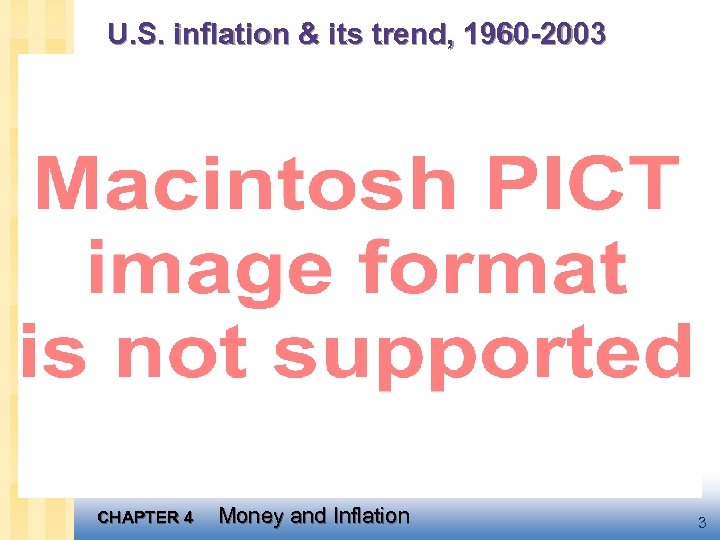U. S. inflation & its trend, 1960 -2003 CHAPTER 4 Money and Inflation 3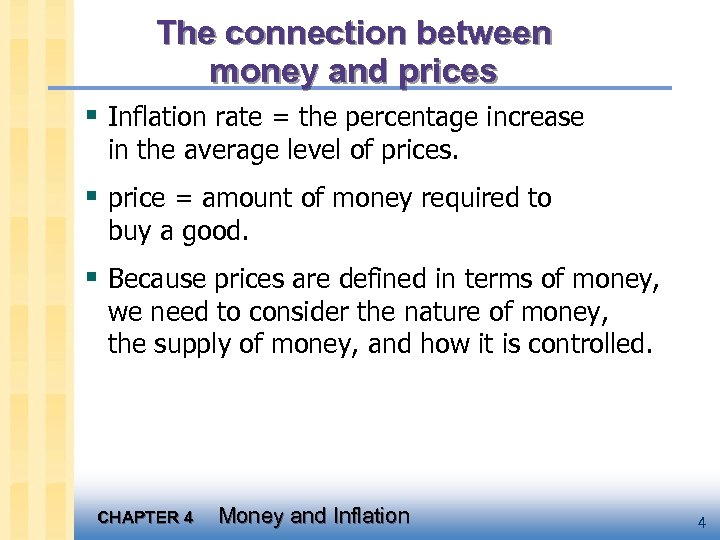The connection between money and prices § Inflation rate = the percentage increase in the average level of prices. § price = amount of money required to buy a good. § Because prices are defined in terms of money, we need to consider the nature of money, the supply of money, and how it is controlled. CHAPTER 4 Money and Inflation 4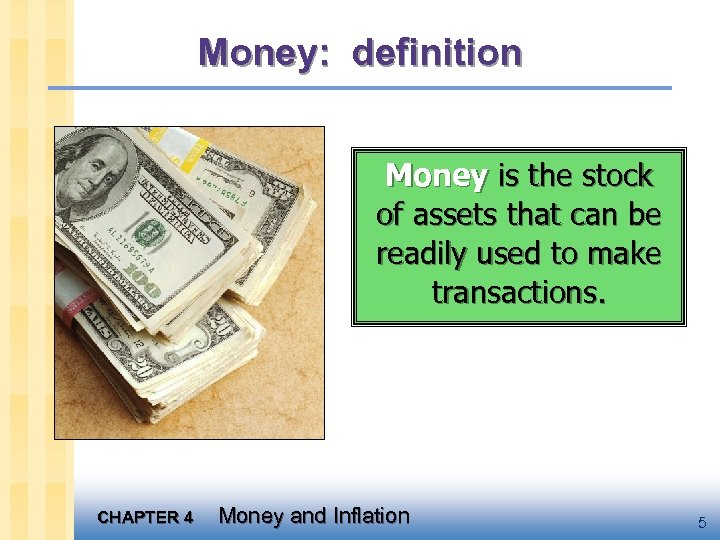Money: definition Money is the stock of assets that can be readily used to make transactions. CHAPTER 4 Money and Inflation 5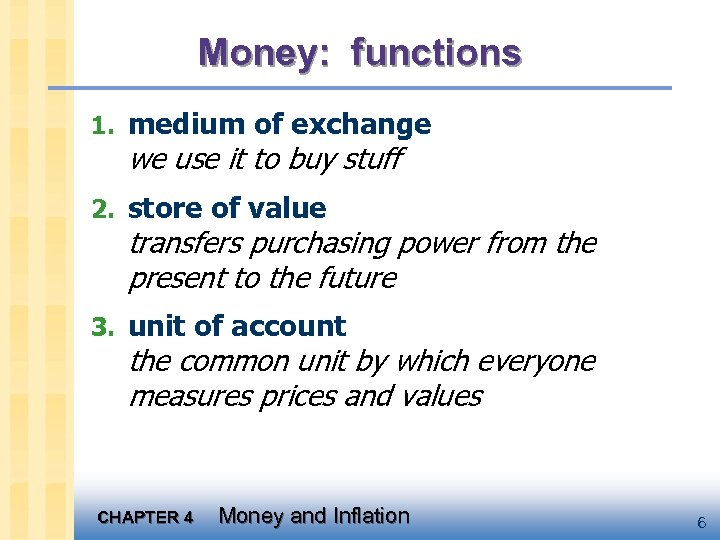Money: functions 1. medium of exchange we use it to buy stuff 2. store of value transfers purchasing power from the present to the future 3. unit of account the common unit by which everyone measures prices and values CHAPTER 4 Money and Inflation 6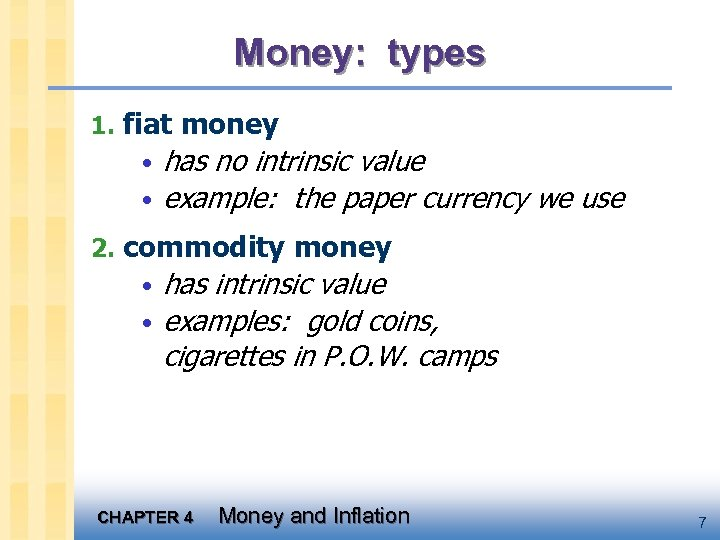Money: types 1. fiat money • has no intrinsic value • example: the paper currency we use 2. commodity money • has intrinsic value • examples: gold coins, cigarettes in P. O. W. camps CHAPTER 4 Money and Inflation 7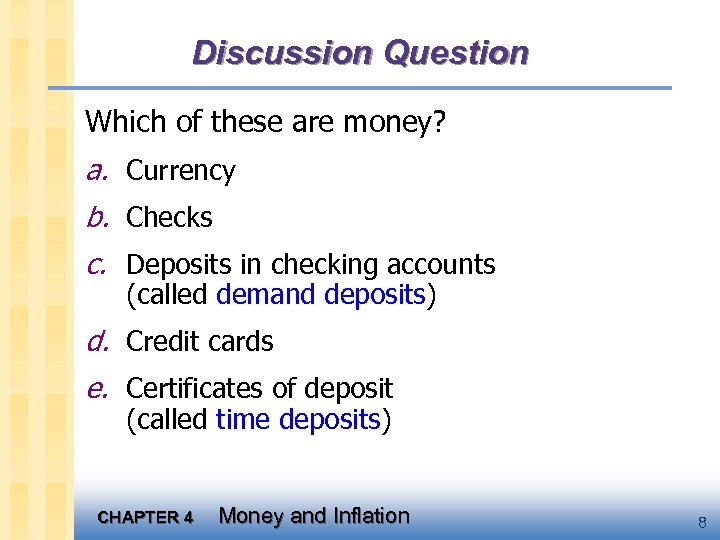Discussion Question Which of these are money? a. Currency b. Checks c. Deposits in checking accounts (called demand deposits) d. Credit cards e. Certificates of deposit (called time deposits) CHAPTER 4 Money and Inflation 8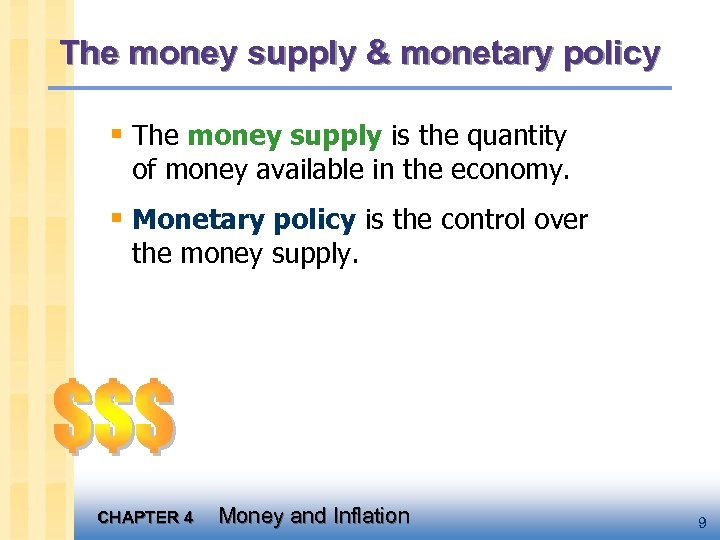The money supply & monetary policy § The money supply is the quantity of money available in the economy. § Monetary policy is the control over the money supply. CHAPTER 4 Money and Inflation 9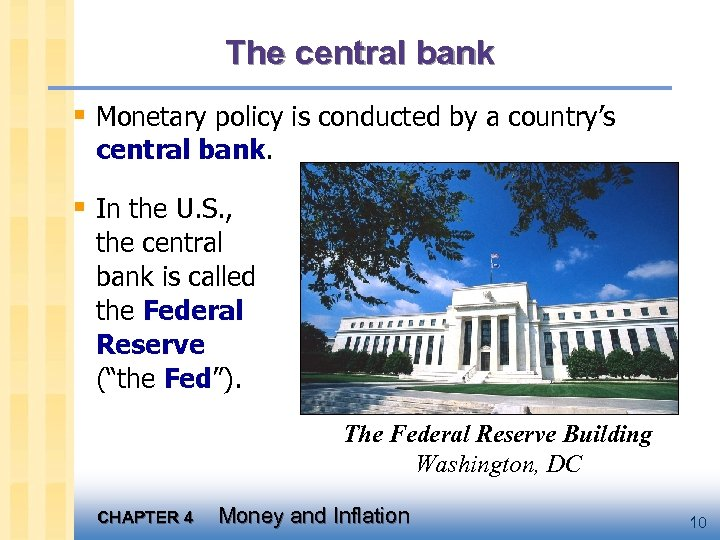The central bank § Monetary policy is conducted by a country’s central bank. § In the U. S. , the central bank is called the Federal Reserve (“the Fed”). The Federal Reserve Building Washington, DC CHAPTER 4 Money and Inflation 10Money supply measures, April 2002 _Symbol Assets included Amount (billions)_ C Currency \$598. 7 M 1 C + demand deposits, travelers’ checks, other checkable deposits M 2 M 1 + small time deposits, savings deposits, money market mutual funds, money market deposit accounts 5480. 1 M 3 M 2 + large time deposits, repurchase agreements, institutional money market mutual fund balances 8054. 4 CHAPTER 4 Money and Inflation 1174. 0 11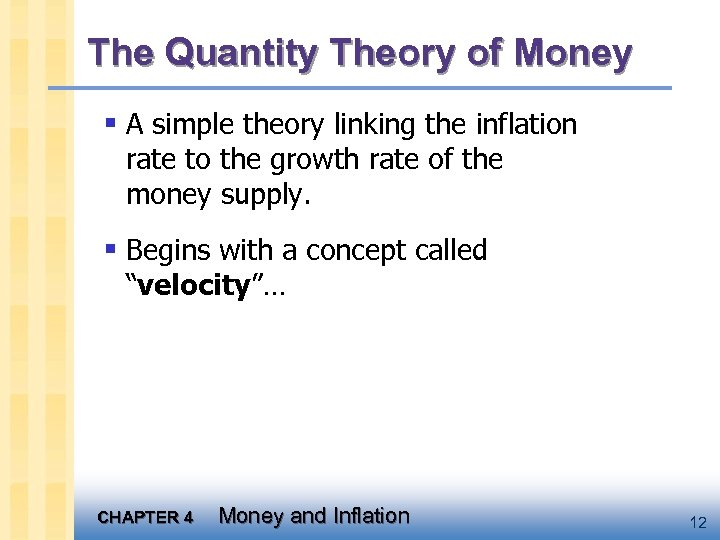The Quantity Theory of Money § A simple theory linking the inflation rate to the growth rate of the money supply. § Begins with a concept called “velocity”… CHAPTER 4 Money and Inflation 12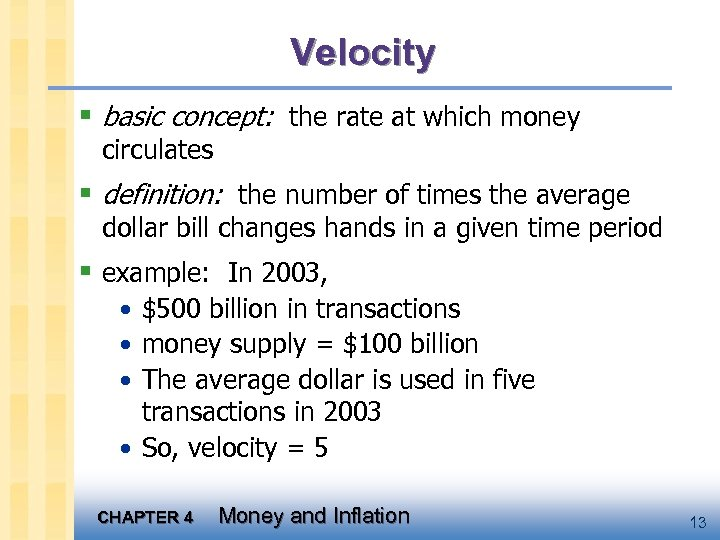Velocity § basic concept: the rate at which money circulates § definition: the number of times the average dollar bill changes hands in a given time period § example: In 2003, • \$500 billion in transactions • money supply = \$100 billion • The average dollar is used in five transactions in 2003 • So, velocity = 5 CHAPTER 4 Money and Inflation 13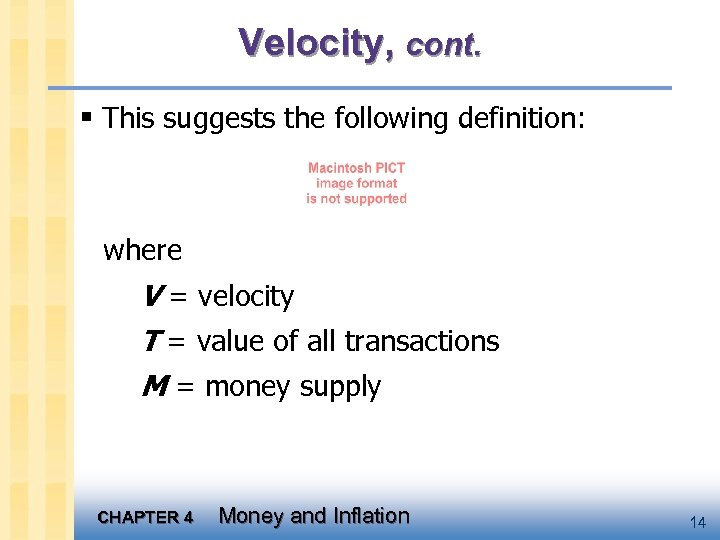Velocity, cont. § This suggests the following definition: where V = velocity T = value of all transactions M = money supply CHAPTER 4 Money and Inflation 14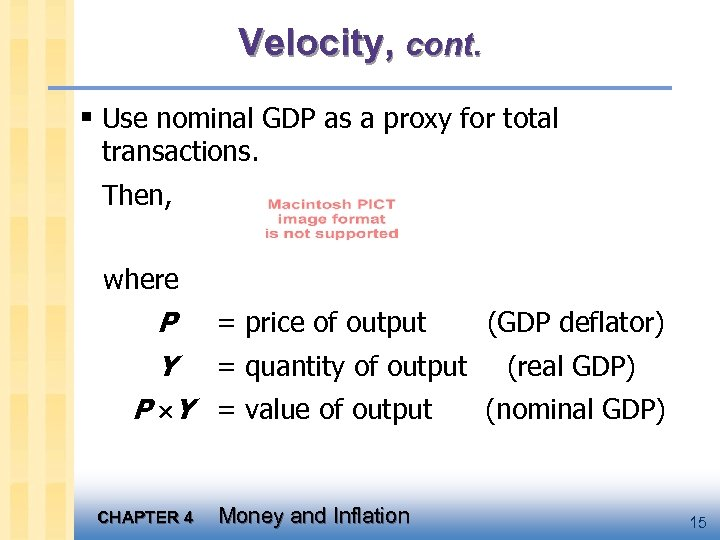Velocity, cont. § Use nominal GDP as a proxy for total transactions. Then, where P = price of output (GDP deflator) Y = quantity of output (real GDP) P Y = value of output (nominal GDP) CHAPTER 4 Money and Inflation 15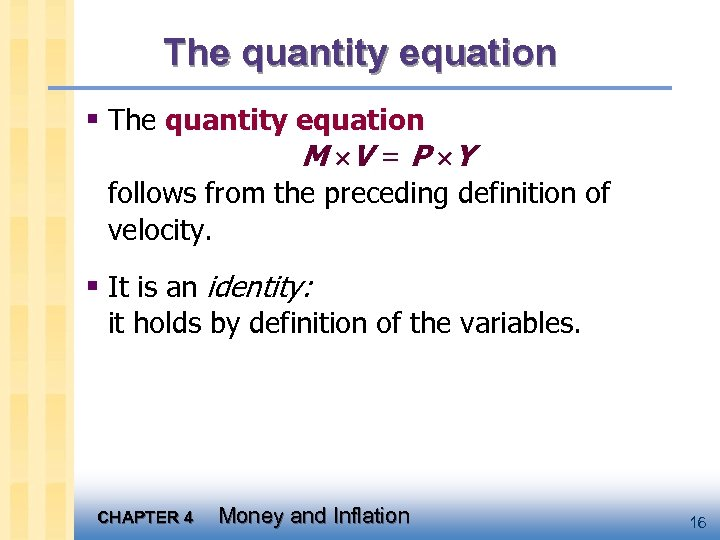The quantity equation § The quantity equation M V = P Y follows from the preceding definition of velocity. § It is an identity: it holds by definition of the variables. CHAPTER 4 Money and Inflation 16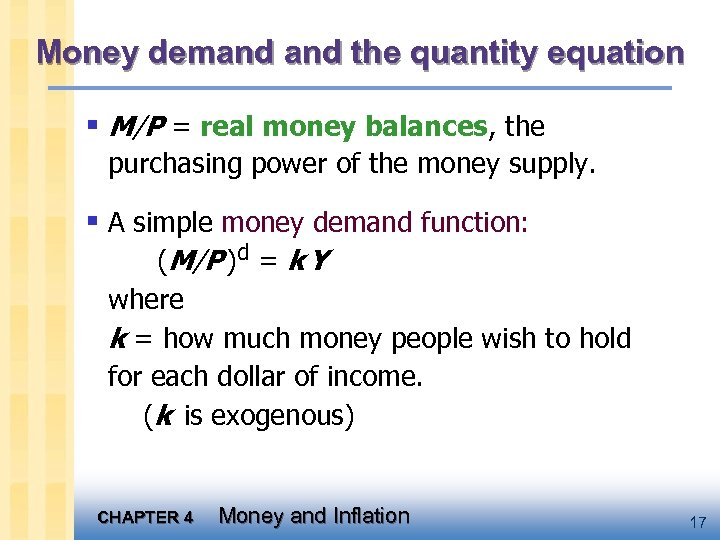Money demand the quantity equation § M/P = real money balances, the purchasing power of the money supply. § A simple money demand function: (M/P )d = k Y where k = how much money people wish to hold for each dollar of income. (k is exogenous) CHAPTER 4 Money and Inflation 17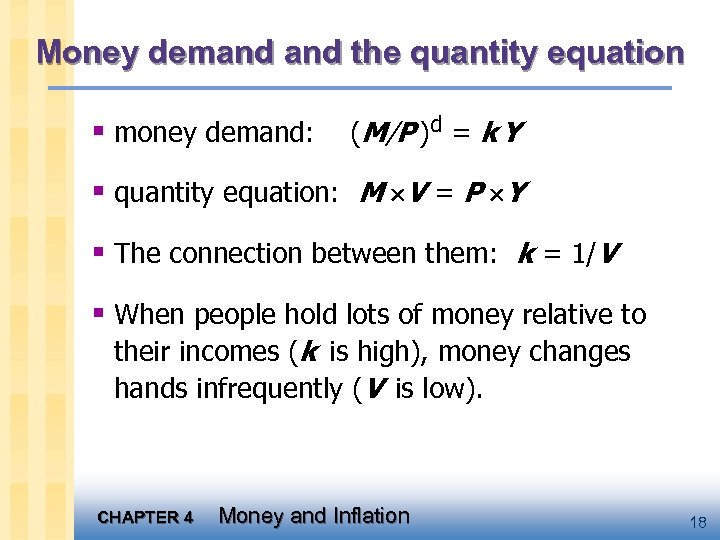Money demand the quantity equation § money demand: (M/P )d = k Y § quantity equation: M V = P Y § The connection between them: k = 1/V § When people hold lots of money relative to their incomes (k is high), money changes hands infrequently (V is low). CHAPTER 4 Money and Inflation 18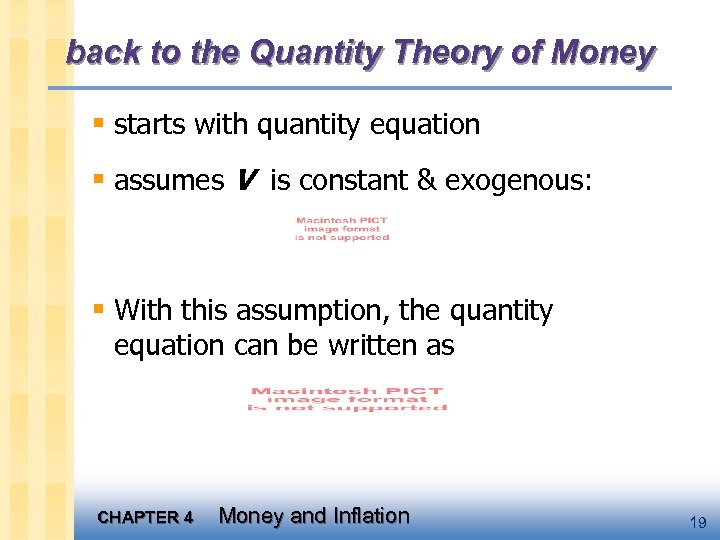back to the Quantity Theory of Money § starts with quantity equation § assumes V is constant & exogenous: § With this assumption, the quantity equation can be written as CHAPTER 4 Money and Inflation 19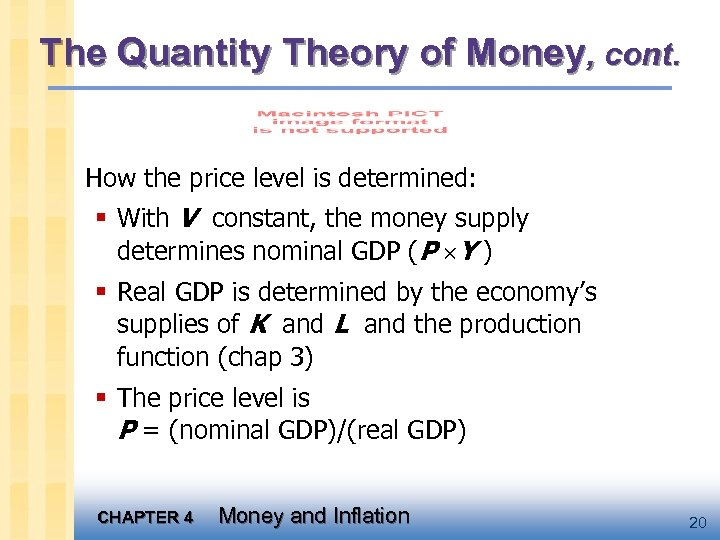The Quantity Theory of Money, cont. How the price level is determined: § With V constant, the money supply determines nominal GDP (P Y ) § Real GDP is determined by the economy’s supplies of K and L and the production function (chap 3) § The price level is P = (nominal GDP)/(real GDP) CHAPTER 4 Money and Inflation 20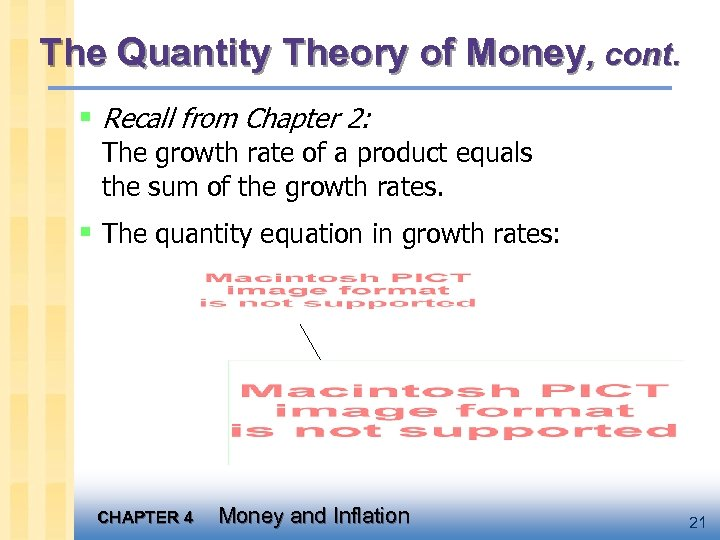The Quantity Theory of Money, cont. § Recall from Chapter 2: The growth rate of a product equals the sum of the growth rates. § The quantity equation in growth rates: CHAPTER 4 Money and Inflation 21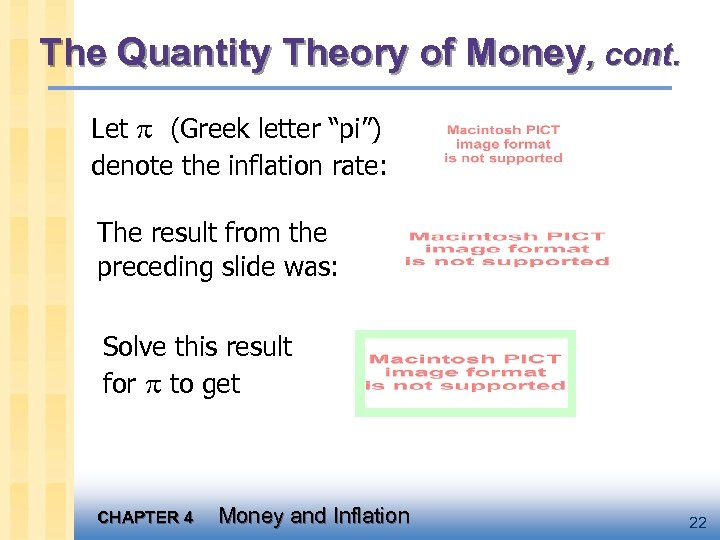The Quantity Theory of Money, cont. Let (Greek letter “pi”) denote the inflation rate: The result from the preceding slide was: Solve this result for to get CHAPTER 4 Money and Inflation 22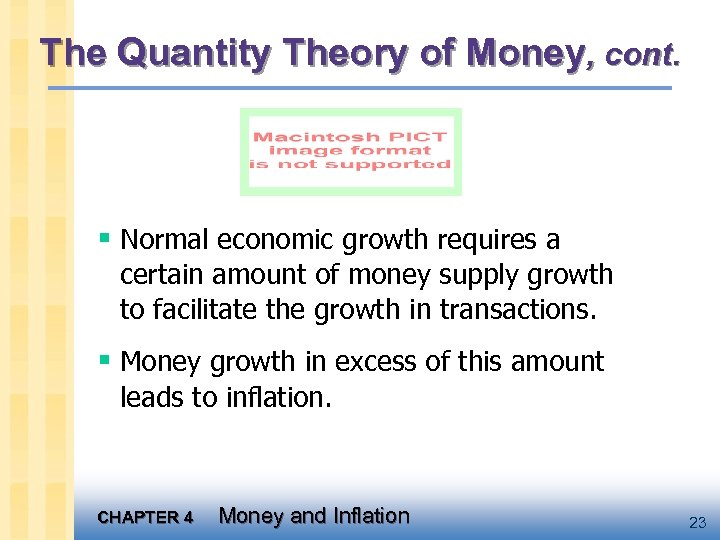The Quantity Theory of Money, cont. § Normal economic growth requires a certain amount of money supply growth to facilitate the growth in transactions. § Money growth in excess of this amount leads to inflation. CHAPTER 4 Money and Inflation 23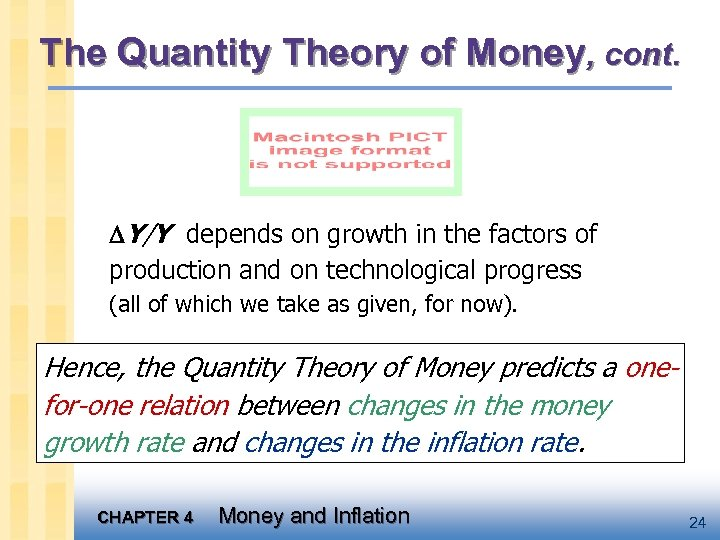The Quantity Theory of Money, cont. Y/Y depends on growth in the factors of production and on technological progress (all of which we take as given, for now). Hence, the Quantity Theory of Money predicts a onefor-one relation between changes in the money growth rate and changes in the inflation rate. CHAPTER 4 Money and Inflation 24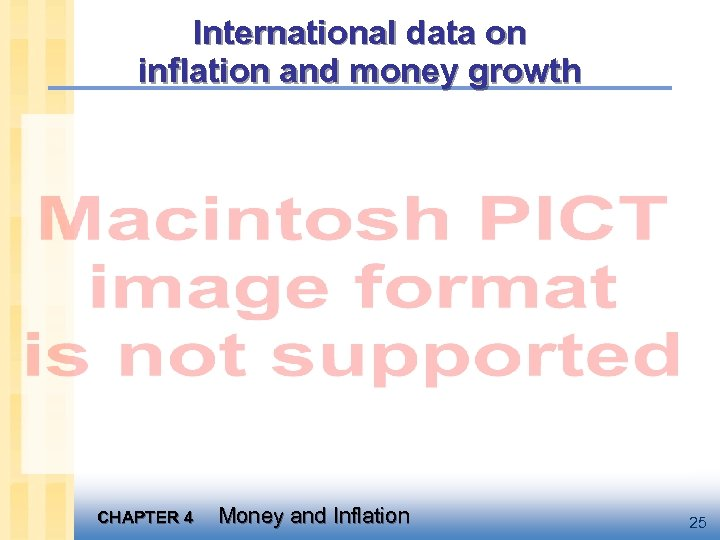International data on inflation and money growth CHAPTER 4 Money and Inflation 25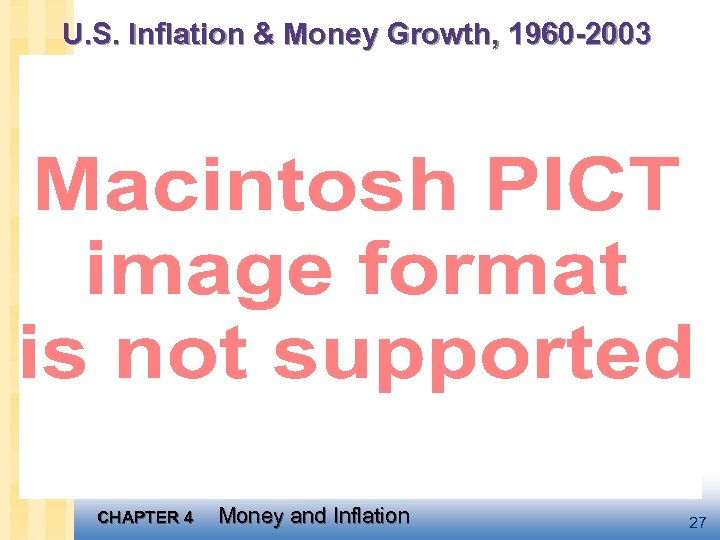U. S. Inflation & Money Growth, 1960 -2003 CHAPTER 4 Money and Inflation 27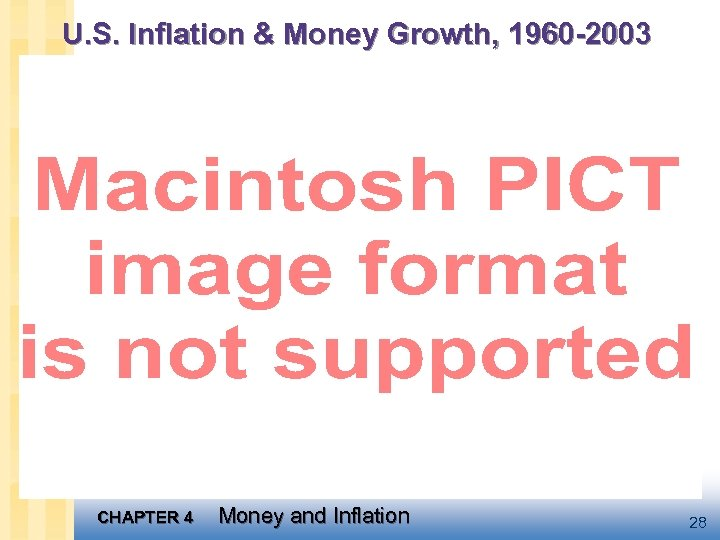U. S. Inflation & Money Growth, 1960 -2003 CHAPTER 4 Money and Inflation 28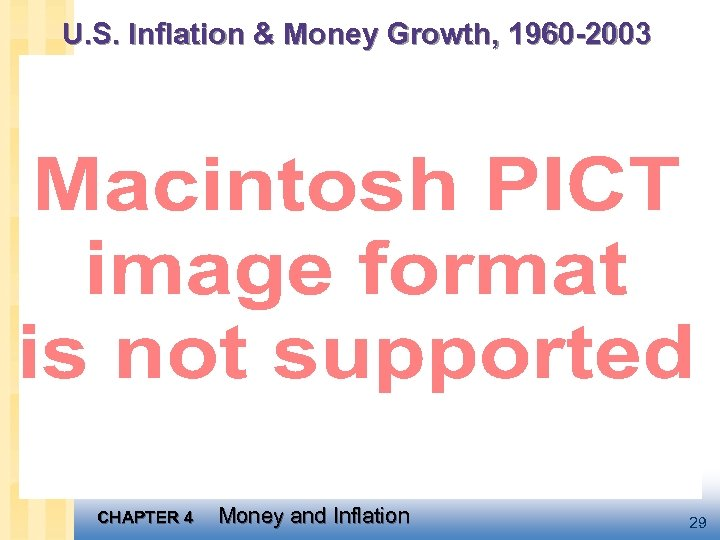U. S. Inflation & Money Growth, 1960 -2003 CHAPTER 4 Money and Inflation 29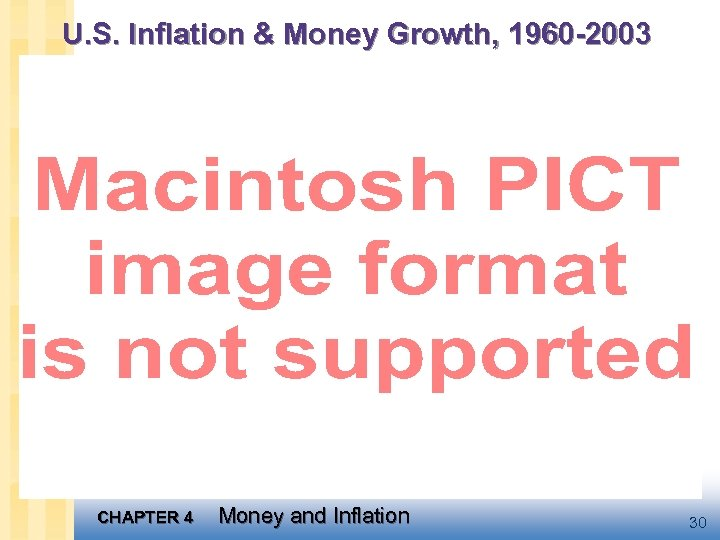U. S. Inflation & Money Growth, 1960 -2003 CHAPTER 4 Money and Inflation 30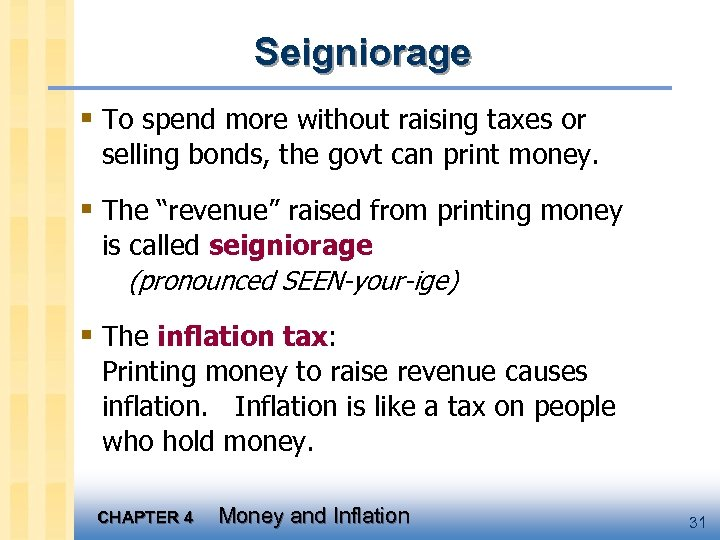Seigniorage § To spend more without raising taxes or selling bonds, the govt can print money. § The “revenue” raised from printing money is called seigniorage (pronounced SEEN-your-ige) § The inflation tax: Printing money to raise revenue causes inflation. Inflation is like a tax on people who hold money. CHAPTER 4 Money and Inflation 31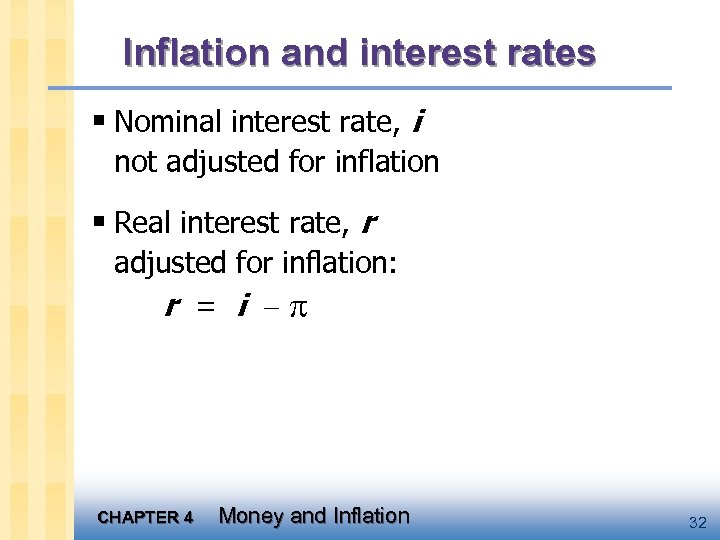Inflation and interest rates § Nominal interest rate, i not adjusted for inflation § Real interest rate, r adjusted for inflation: r = i CHAPTER 4 Money and Inflation 32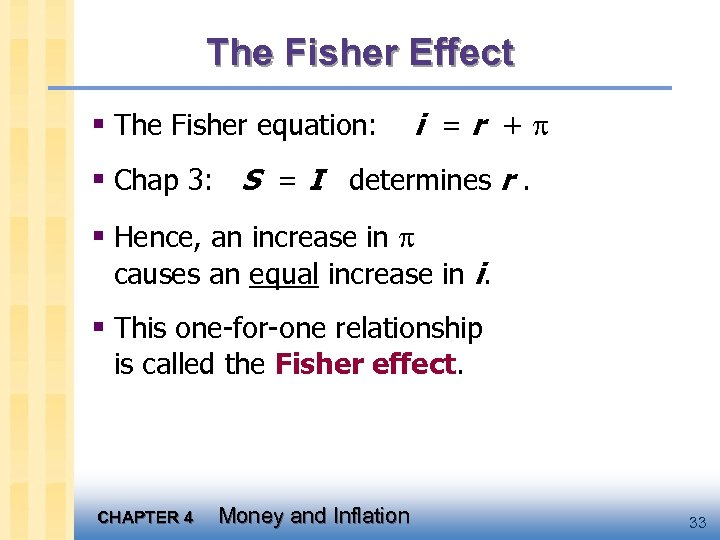The Fisher Effect § The Fisher equation: i =r + § Chap 3: S = I determines r. § Hence, an increase in causes an equal increase in i. § This one-for-one relationship is called the Fisher effect. CHAPTER 4 Money and Inflation 33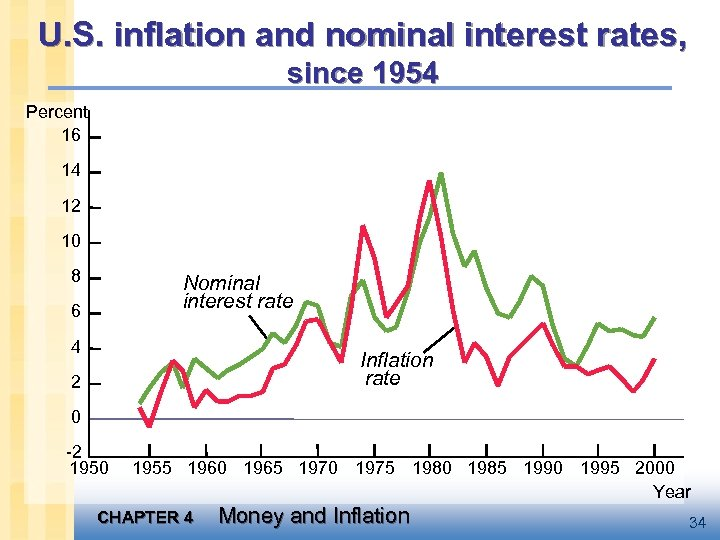U. S. inflation and nominal interest rates, since 1954 Percent 16 14 12 10 8 Nominal interest rate 6 4 Inflation rate 2 0 -2 1950 1955 1960 1965 1970 1975 1980 1985 1990 1995 2000 Year CHAPTER 4 Money and Inflation 34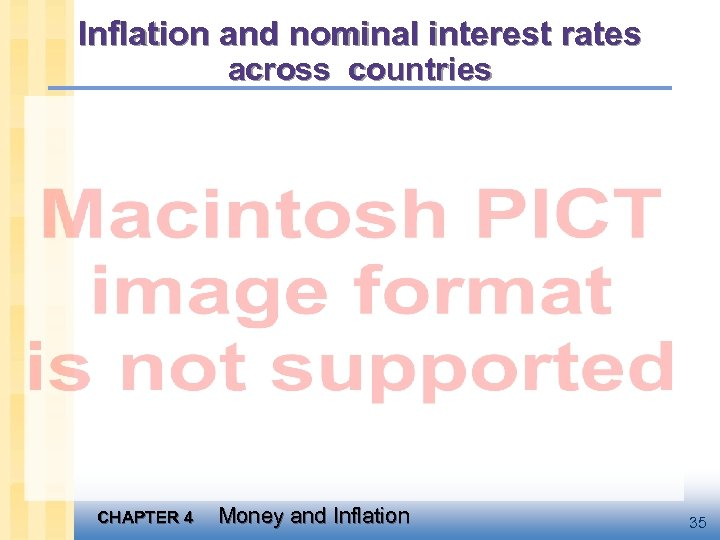Inflation and nominal interest rates across countries CHAPTER 4 Money and Inflation 35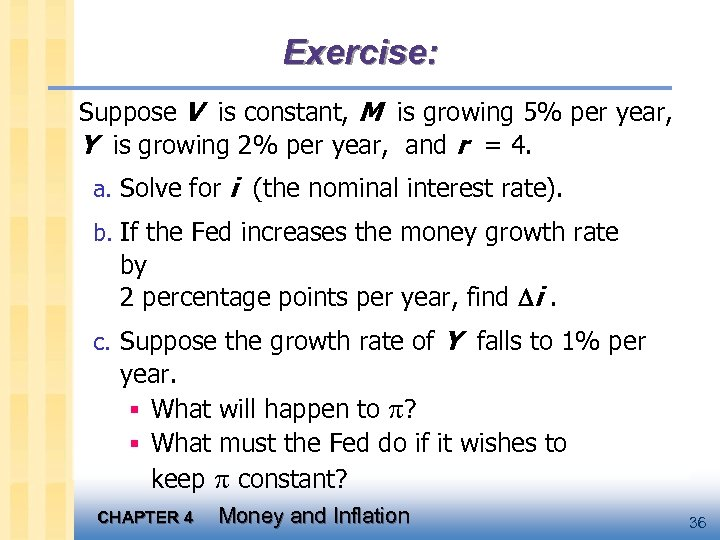Exercise: Suppose V is constant, M is growing 5% per year, Y is growing 2% per year, and r = 4. a. Solve for i (the nominal interest rate). b. If the Fed increases the money growth rate by 2 percentage points per year, find i. c. Suppose the growth rate of Y falls to 1% per year. § What will happen to ? § What must the Fed do if it wishes to keep constant? CHAPTER 4 Money and Inflation 36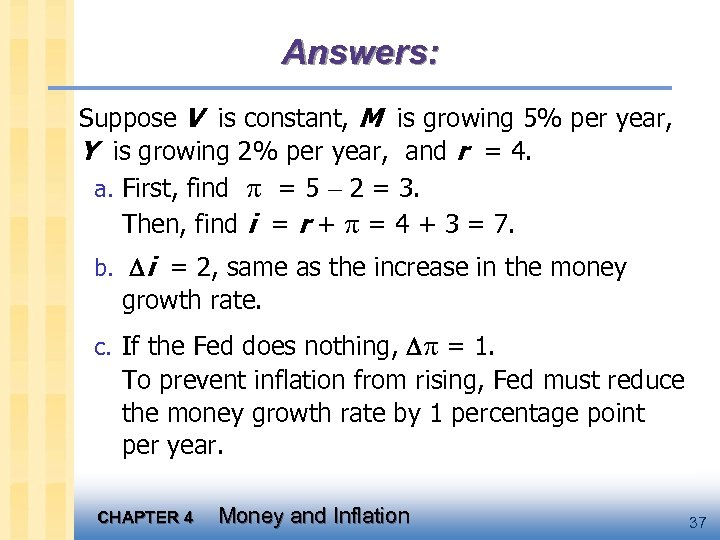Answers: Suppose V is constant, M is growing 5% per year, Y is growing 2% per year, and r = 4. a. First, find = 5 2 = 3. Then, find i = r + = 4 + 3 = 7. b. i = 2, same as the increase in the money growth rate. c. If the Fed does nothing, = 1. To prevent inflation from rising, Fed must reduce the money growth rate by 1 percentage point per year. CHAPTER 4 Money and Inflation 37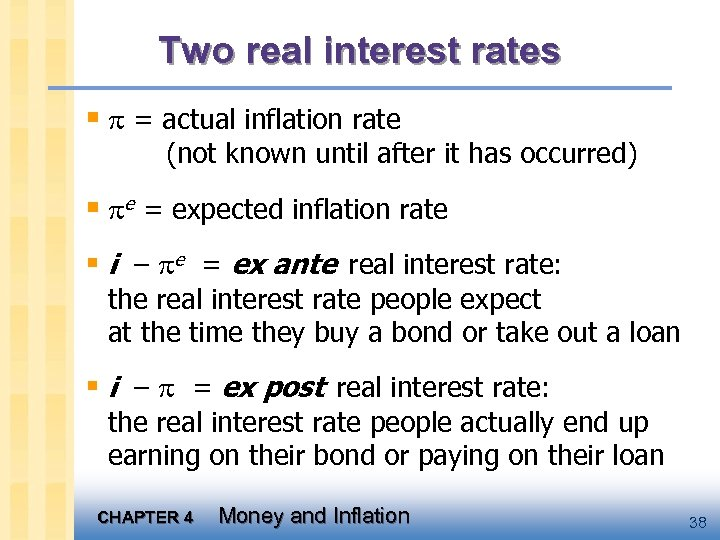Two real interest rates § = actual inflation rate (not known until after it has occurred) § e = expected inflation rate § i – e = ex ante real interest rate: the real interest rate people expect at the time they buy a bond or take out a loan § i – = ex post real interest rate: the real interest rate people actually end up earning on their bond or paying on their loan CHAPTER 4 Money and Inflation 38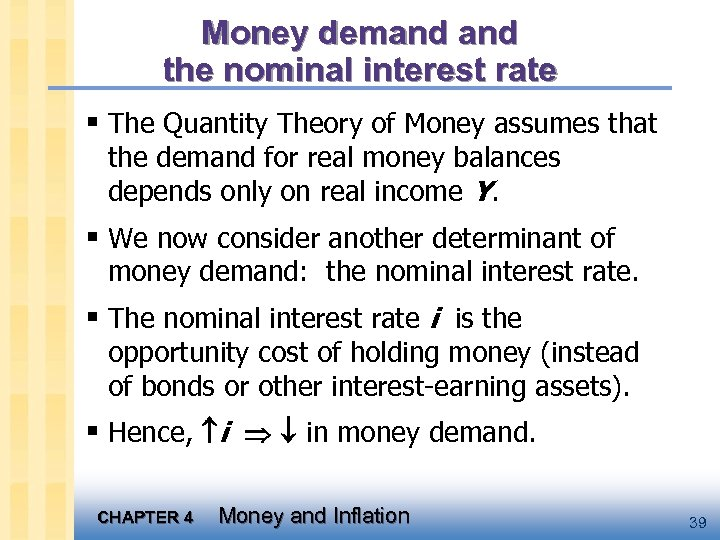Money demand the nominal interest rate § The Quantity Theory of Money assumes that the demand for real money balances depends only on real income Y. § We now consider another determinant of money demand: the nominal interest rate. § The nominal interest rate i is the opportunity cost of holding money (instead of bonds or other interest-earning assets). § Hence, i in money demand. CHAPTER 4 Money and Inflation 39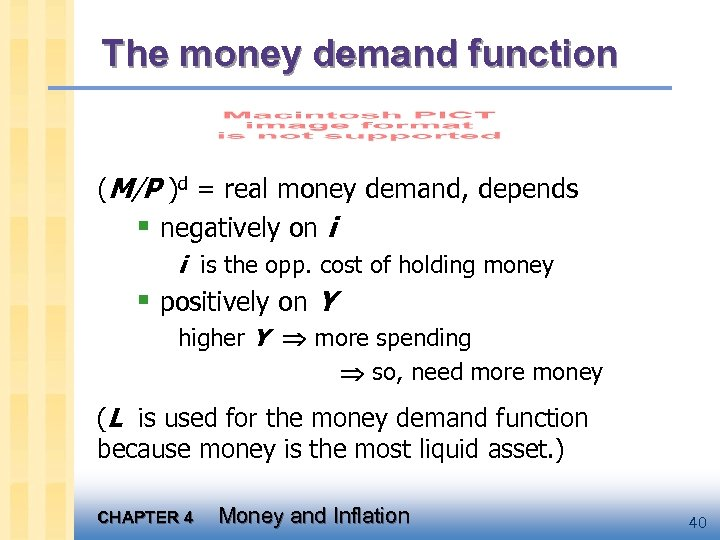The money demand function (M/P )d = real money demand, depends § negatively on i i is the opp. cost of holding money § positively on Y higher Y more spending so, need more money (L is used for the money demand function because money is the most liquid asset. ) CHAPTER 4 Money and Inflation 40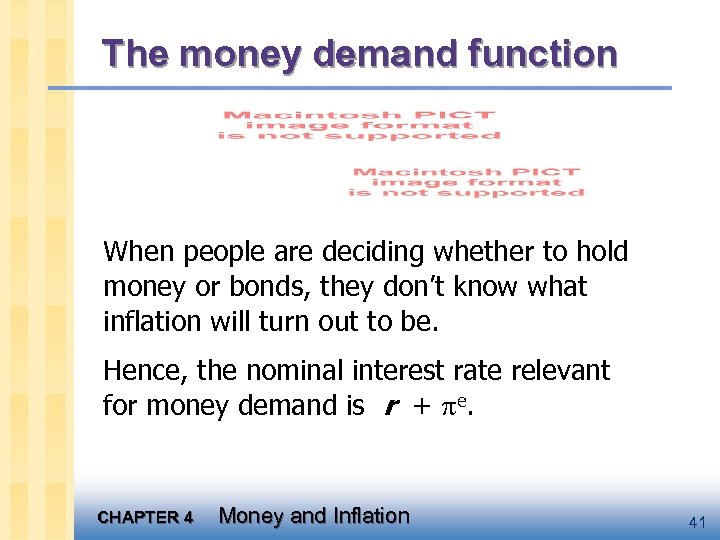The money demand function When people are deciding whether to hold money or bonds, they don’t know what inflation will turn out to be. Hence, the nominal interest rate relevant for money demand is r + e. CHAPTER 4 Money and Inflation 41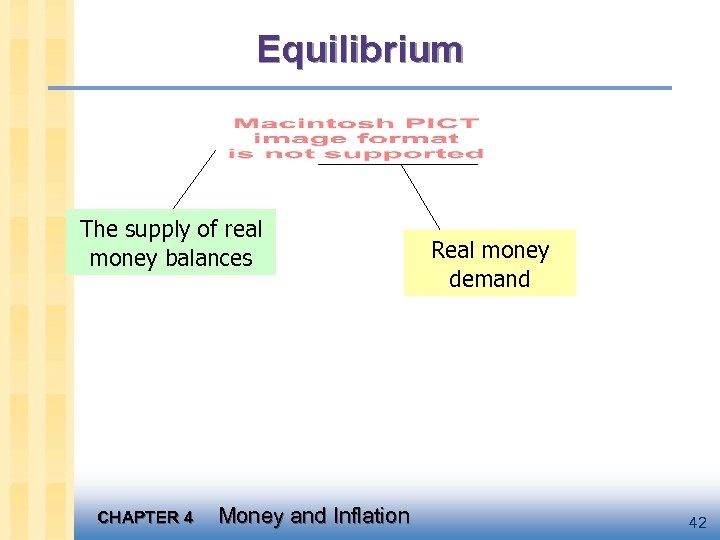Equilibrium The supply of real money balances CHAPTER 4 Money and Inflation Real money demand 42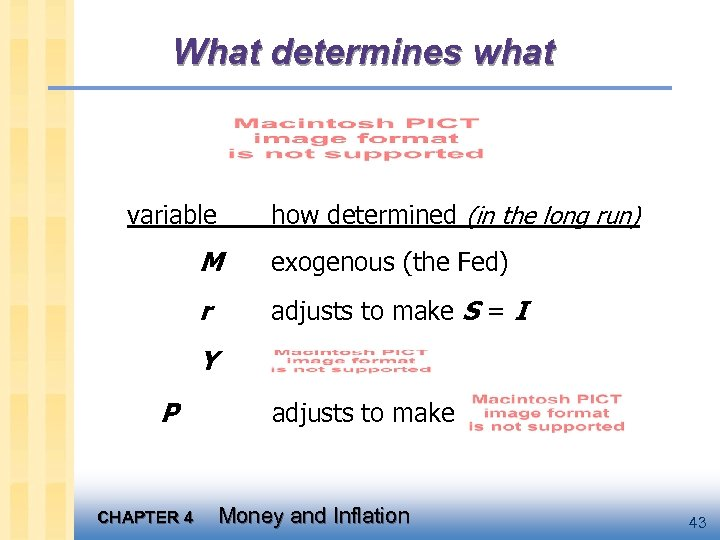What determines what variable how determined (in the long run) M exogenous (the Fed) r adjusts to make S = I Y P CHAPTER 4 adjusts to make Money and Inflation 43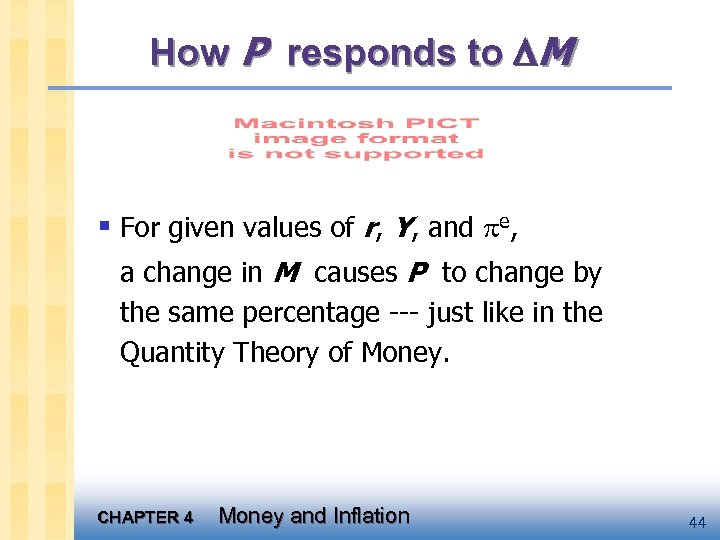How P responds to M § For given values of r, Y, and e, a change in M causes P to change by the same percentage --- just like in the Quantity Theory of Money. CHAPTER 4 Money and Inflation 44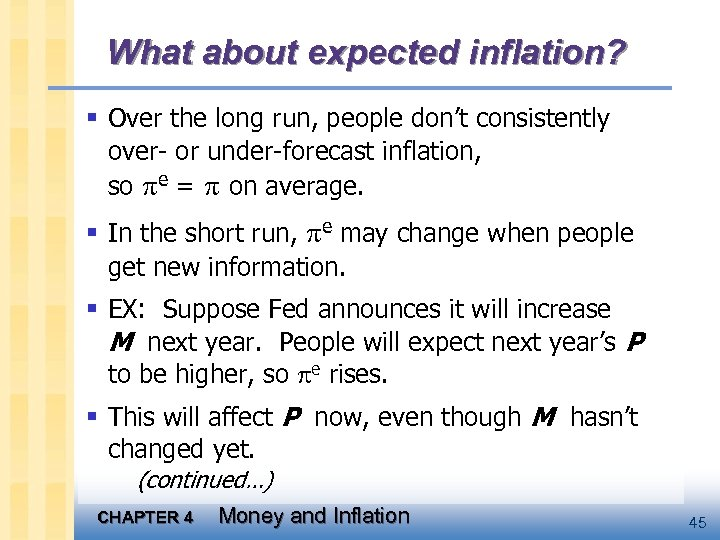What about expected inflation? § Over the long run, people don’t consistently over- or under-forecast inflation, so e = on average. § In the short run, e may change when people get new information. § EX: Suppose Fed announces it will increase M next year. People will expect next year’s P to be higher, so e rises. § This will affect P now, even though M hasn’t changed yet. (continued…) CHAPTER 4 Money and Inflation 45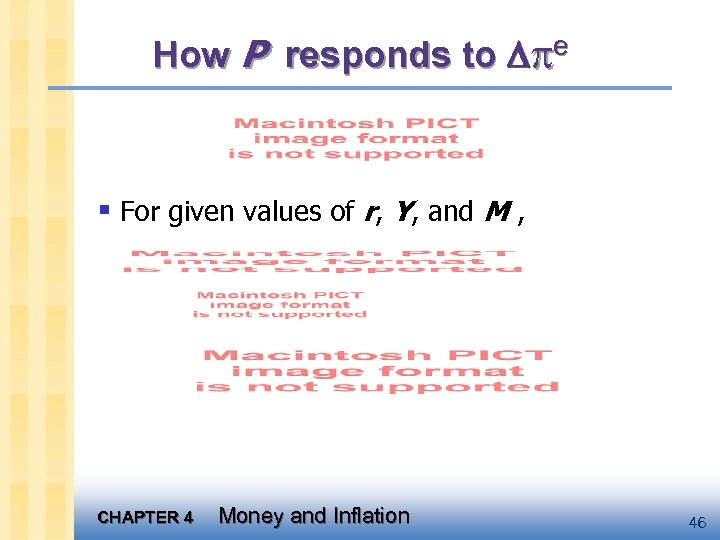How P responds to e § For given values of r, Y, and M , CHAPTER 4 Money and Inflation 46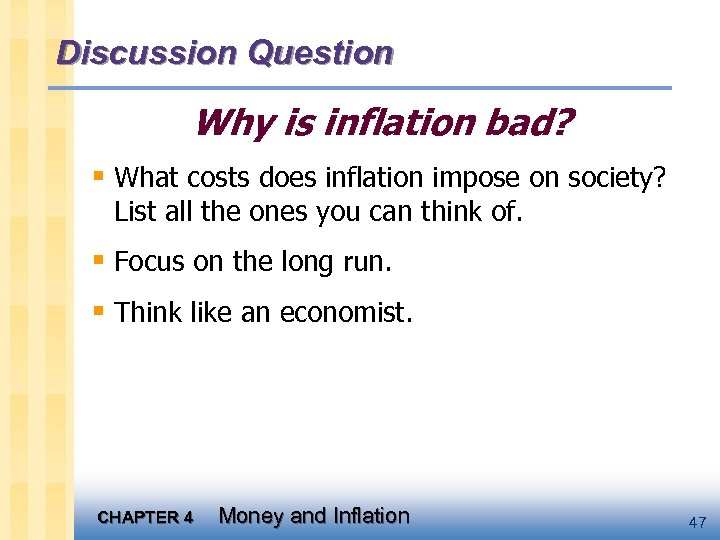Discussion Question Why is inflation bad? § What costs does inflation impose on society? List all the ones you can think of. § Focus on the long run. § Think like an economist. CHAPTER 4 Money and Inflation 47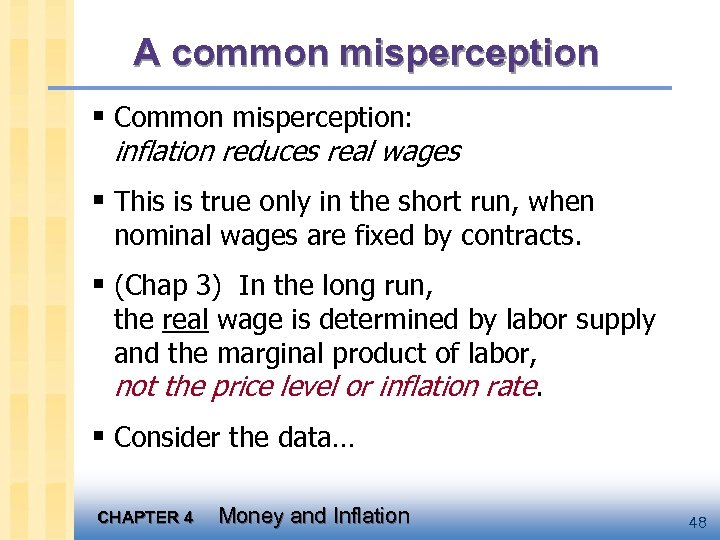A common misperception § Common misperception: inflation reduces real wages § This is true only in the short run, when nominal wages are fixed by contracts. § (Chap 3) In the long run, the real wage is determined by labor supply and the marginal product of labor, not the price level or inflation rate. § Consider the data… CHAPTER 4 Money and Inflation 48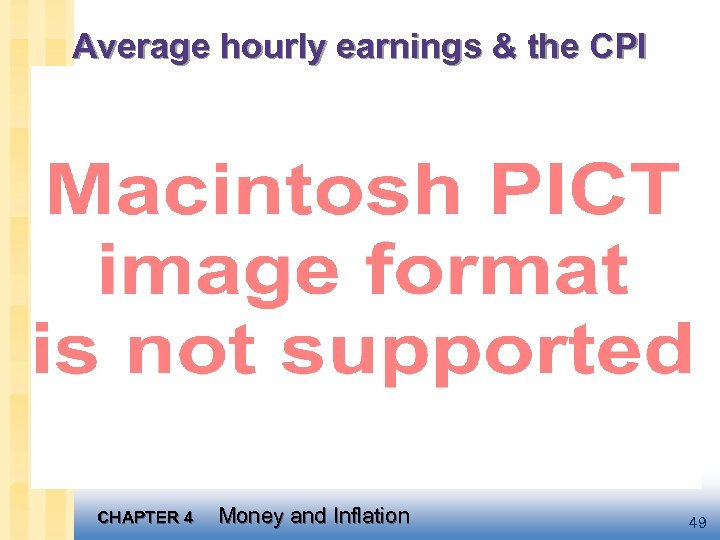Average hourly earnings & the CPI CHAPTER 4 Money and Inflation 49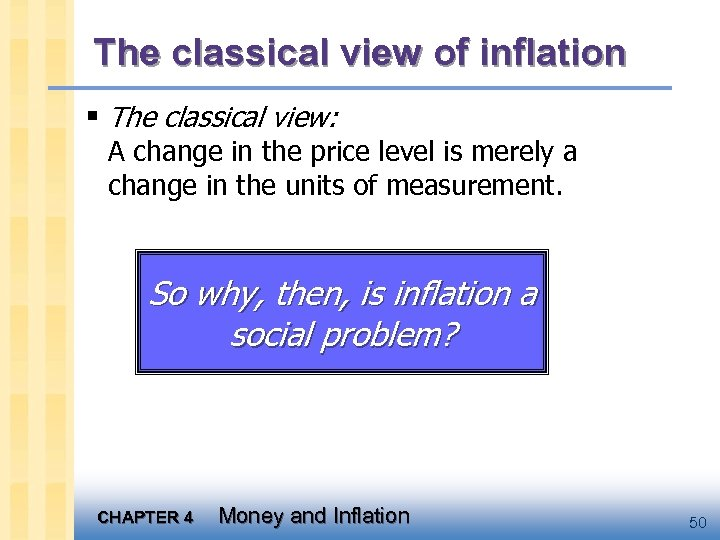The classical view of inflation § The classical view: A change in the price level is merely a change in the units of measurement. So why, then, is inflation a social problem? CHAPTER 4 Money and Inflation 50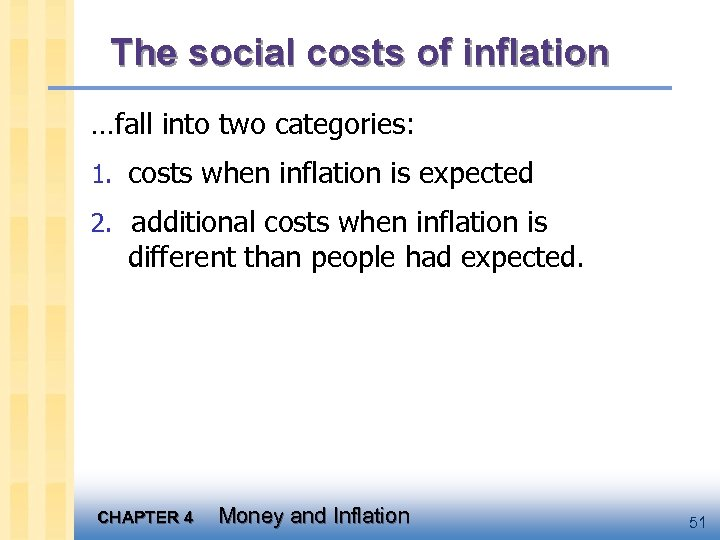The social costs of inflation …fall into two categories: 1. costs when inflation is expected 2. additional costs when inflation is different than people had expected. CHAPTER 4 Money and Inflation 51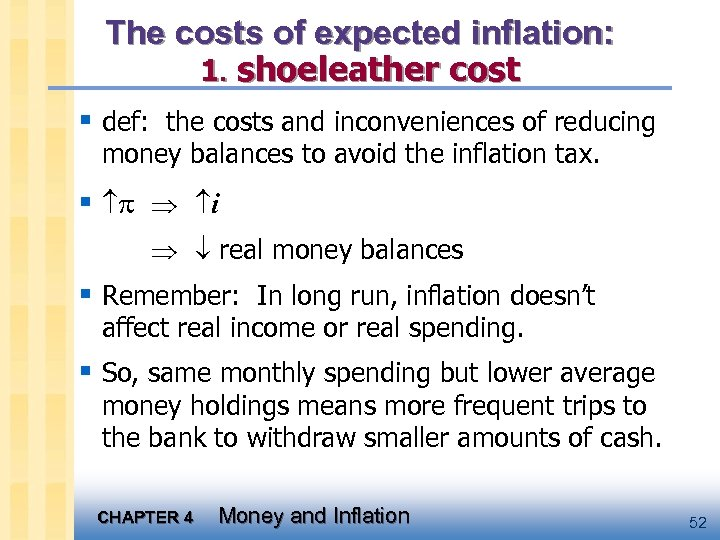The costs of expected inflation: 1. shoeleather cost § def: the costs and inconveniences of reducing money balances to avoid the inflation tax. § i real money balances § Remember: In long run, inflation doesn’t affect real income or real spending. § So, same monthly spending but lower average money holdings means more frequent trips to the bank to withdraw smaller amounts of cash. CHAPTER 4 Money and Inflation 52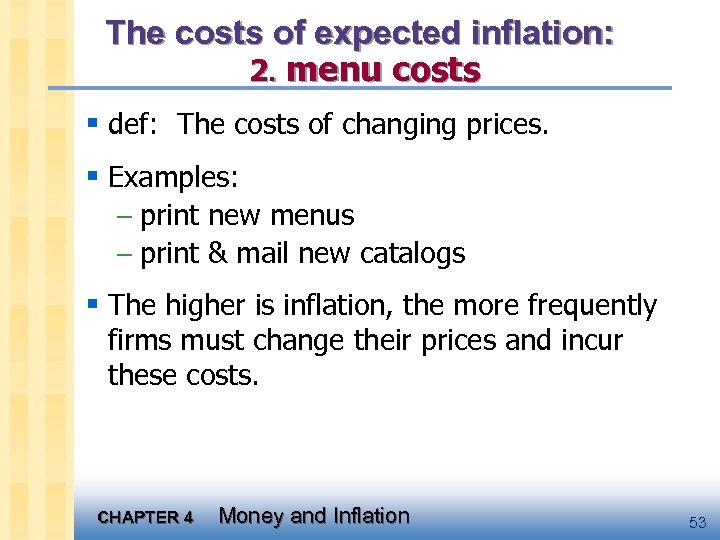The costs of expected inflation: 2. menu costs § def: The costs of changing prices. § Examples: – print new menus – print & mail new catalogs § The higher is inflation, the more frequently firms must change their prices and incur these costs. CHAPTER 4 Money and Inflation 53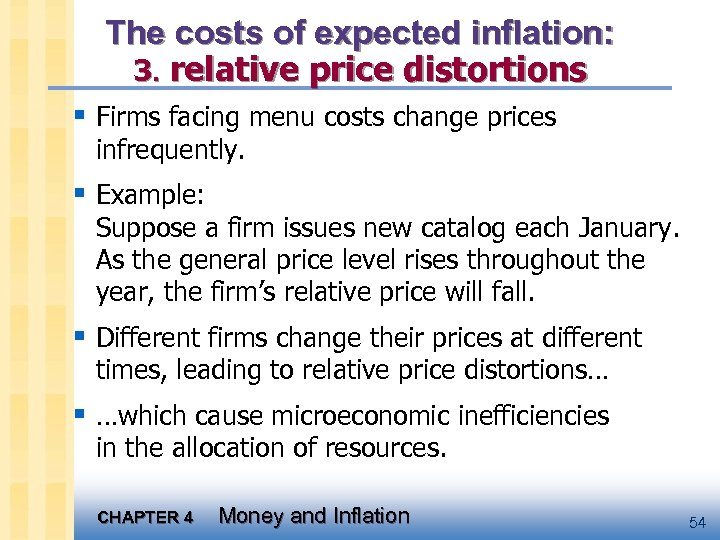The costs of expected inflation: 3. relative price distortions § Firms facing menu costs change prices infrequently. § Example: Suppose a firm issues new catalog each January. As the general price level rises throughout the year, the firm’s relative price will fall. § Different firms change their prices at different times, leading to relative price distortions… § …which cause microeconomic inefficiencies in the allocation of resources. CHAPTER 4 Money and Inflation 54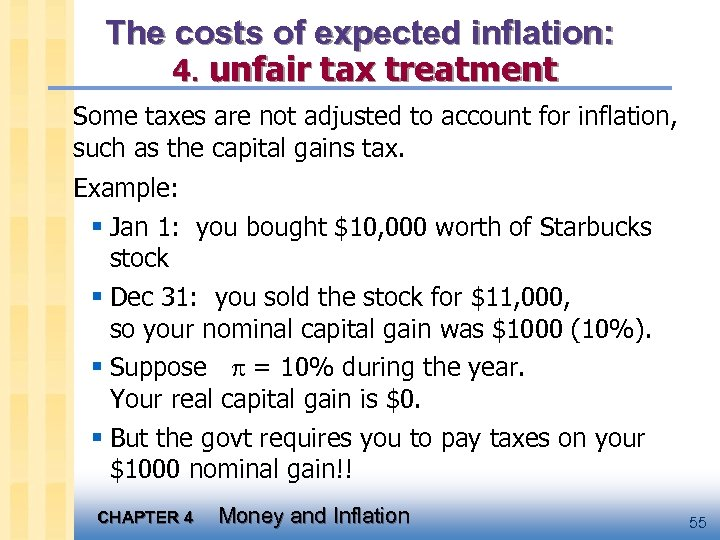The costs of expected inflation: 4. unfair tax treatment Some taxes are not adjusted to account for inflation, such as the capital gains tax. Example: § Jan 1: you bought \$10, 000 worth of Starbucks stock § Dec 31: you sold the stock for \$11, 000, so your nominal capital gain was \$1000 (10%). § Suppose = 10% during the year. Your real capital gain is \$0. § But the govt requires you to pay taxes on your \$1000 nominal gain!! CHAPTER 4 Money and Inflation 55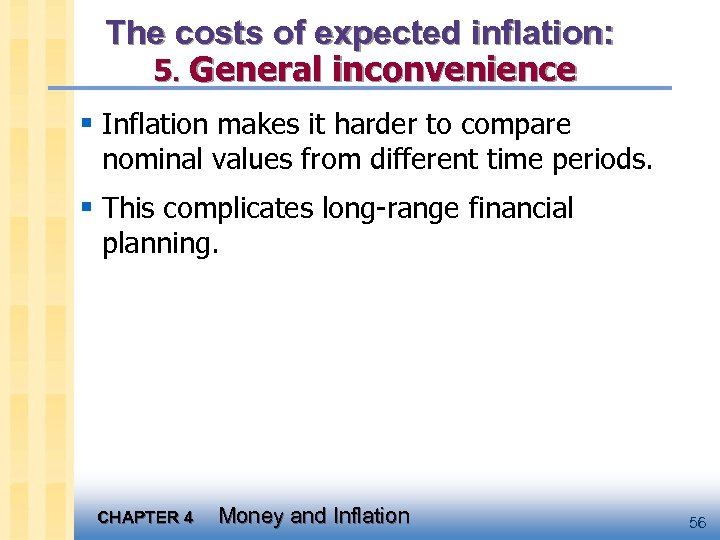The costs of expected inflation: 5. General inconvenience § Inflation makes it harder to compare nominal values from different time periods. § This complicates long-range financial planning. CHAPTER 4 Money and Inflation 56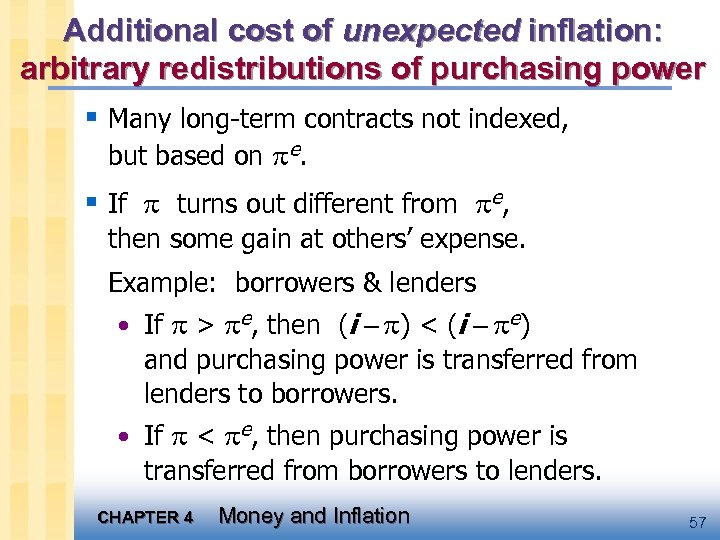Additional cost of unexpected inflation: arbitrary redistributions of purchasing power § Many long-term contracts not indexed, but based on e. § If turns out different from e, then some gain at others’ expense. Example: borrowers & lenders • If > e, then (i ) < (i e) and purchasing power is transferred from lenders to borrowers. • If < e, then purchasing power is transferred from borrowers to lenders. CHAPTER 4 Money and Inflation 57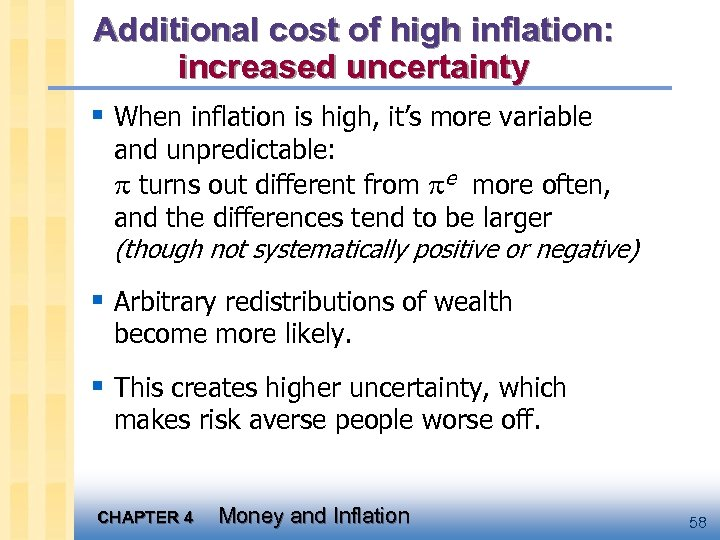Additional cost of high inflation: increased uncertainty § When inflation is high, it’s more variable and unpredictable: turns out different from e more often, and the differences tend to be larger (though not systematically positive or negative) § Arbitrary redistributions of wealth become more likely. § This creates higher uncertainty, which makes risk averse people worse off. CHAPTER 4 Money and Inflation 58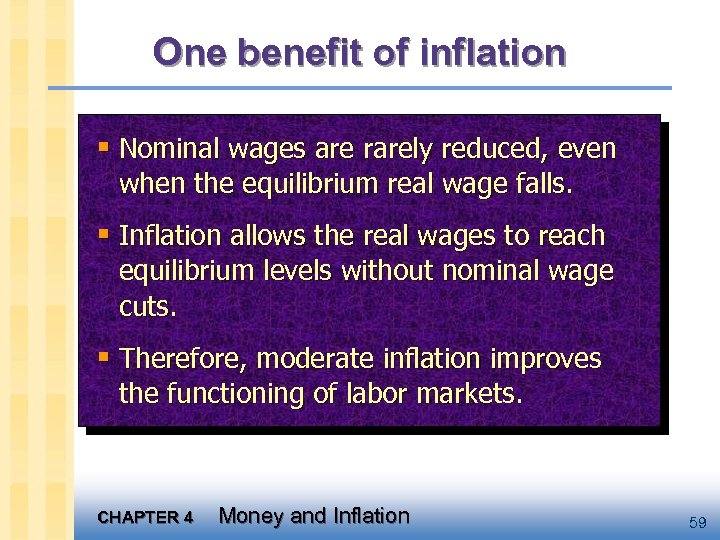One benefit of inflation § Nominal wages are rarely reduced, even when the equilibrium real wage falls. § Inflation allows the real wages to reach equilibrium levels without nominal wage cuts. § Therefore, moderate inflation improves the functioning of labor markets. CHAPTER 4 Money and Inflation 59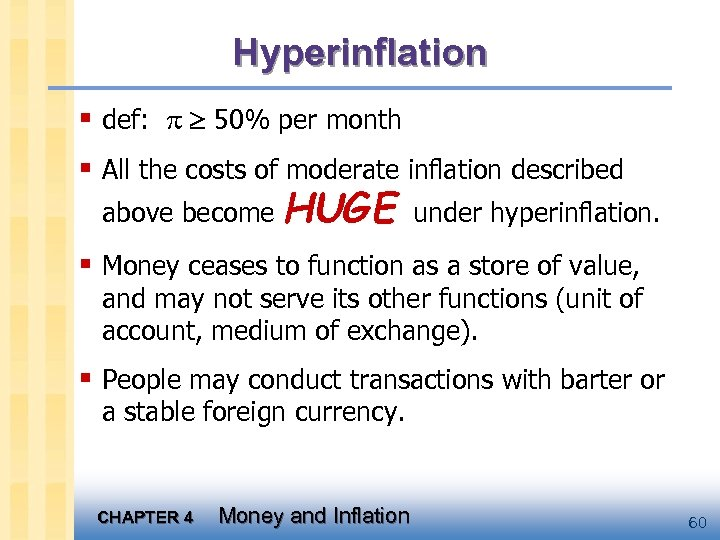Hyperinflation § def: 50% per month § All the costs of moderate inflation described above become HUGE under hyperinflation. § Money ceases to function as a store of value, and may not serve its other functions (unit of account, medium of exchange). § People may conduct transactions with barter or a stable foreign currency. CHAPTER 4 Money and Inflation 60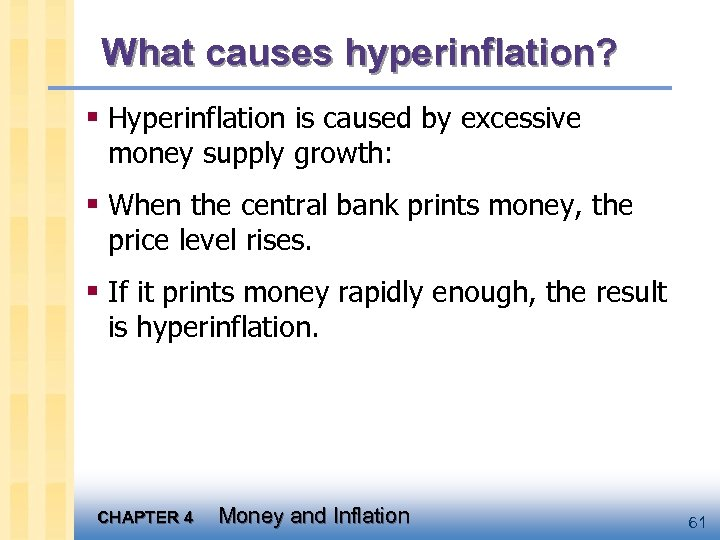What causes hyperinflation? § Hyperinflation is caused by excessive money supply growth: § When the central bank prints money, the price level rises. § If it prints money rapidly enough, the result is hyperinflation. CHAPTER 4 Money and Inflation 61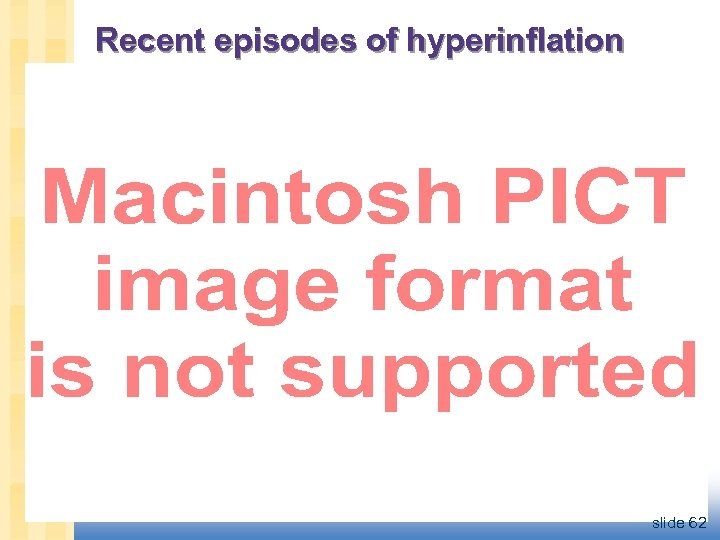Recent episodes of hyperinflation slide 62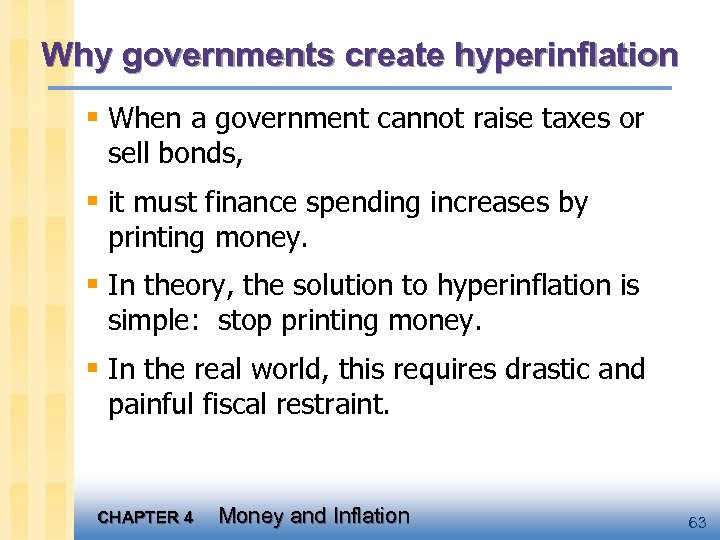Why governments create hyperinflation § When a government cannot raise taxes or sell bonds, § it must finance spending increases by printing money. § In theory, the solution to hyperinflation is simple: stop printing money. § In the real world, this requires drastic and painful fiscal restraint. CHAPTER 4 Money and Inflation 63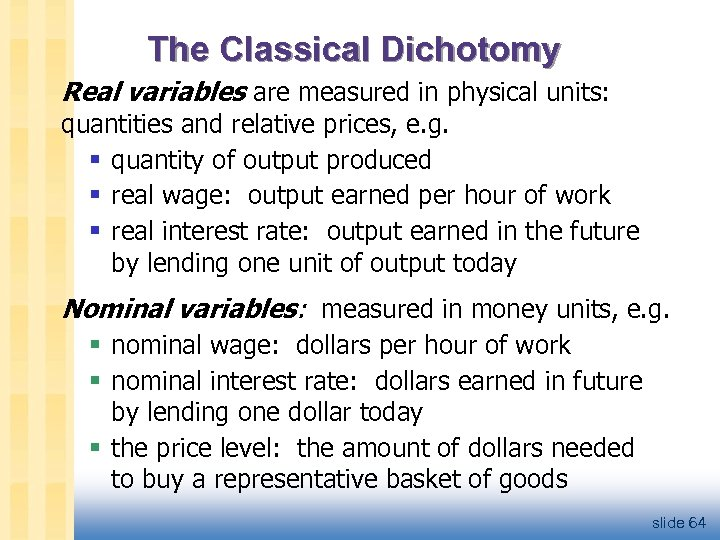The Classical Dichotomy Real variables are measured in physical units: quantities and relative prices, e. g. § quantity of output produced § real wage: output earned per hour of work § real interest rate: output earned in the future by lending one unit of output today Nominal variables: measured in money units, e. g. § nominal wage: dollars per hour of work § nominal interest rate: dollars earned in future by lending one dollar today § the price level: the amount of dollars needed to buy a representative basket of goods slide 64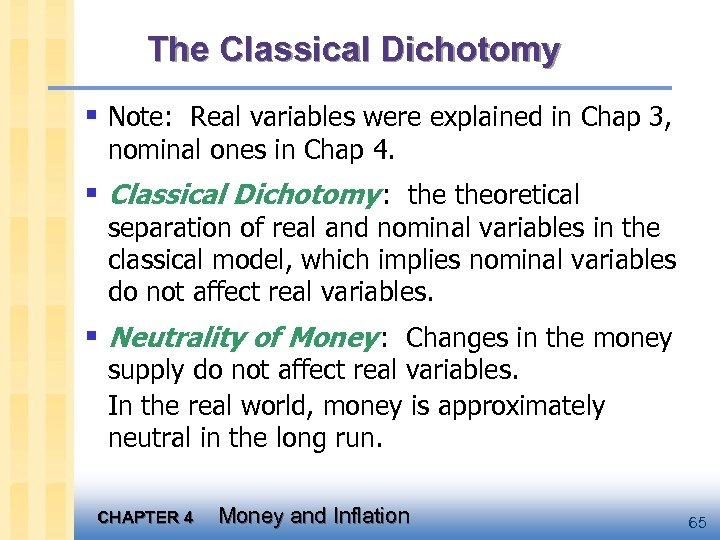The Classical Dichotomy § Note: Real variables were explained in Chap 3, nominal ones in Chap 4. § Classical Dichotomy : theoretical separation of real and nominal variables in the classical model, which implies nominal variables do not affect real variables. § Neutrality of Money : Changes in the money supply do not affect real variables. In the real world, money is approximately neutral in the long run. CHAPTER 4 Money and Inflation 65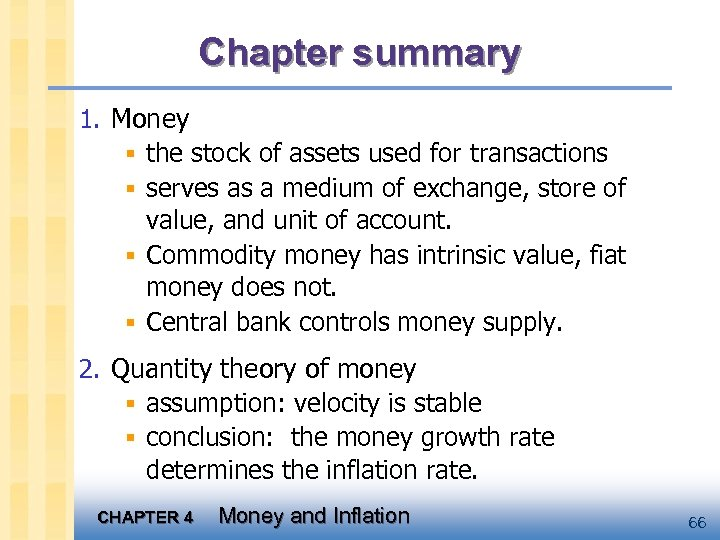Chapter summary 1. Money § the stock of assets used for transactions § serves as a medium of exchange, store of value, and unit of account. § Commodity money has intrinsic value, fiat money does not. § Central bank controls money supply. 2. Quantity theory of money § assumption: velocity is stable § conclusion: the money growth rate determines the inflation rate. CHAPTER 4 Money and Inflation 66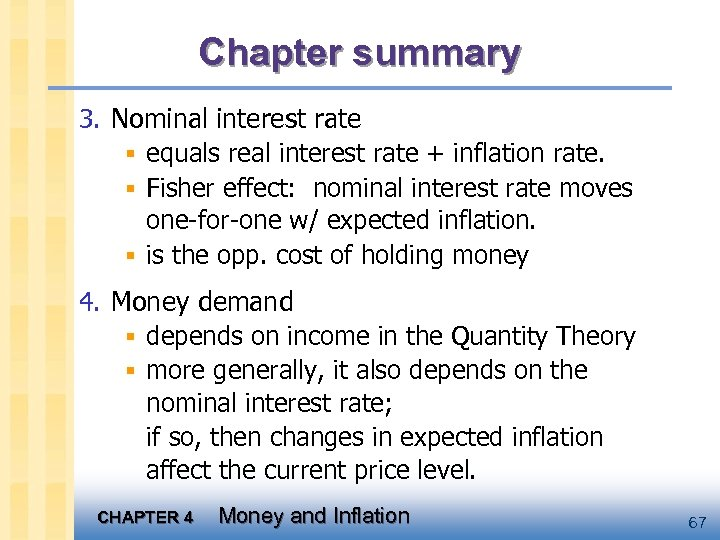Chapter summary 3. Nominal interest rate § equals real interest rate + inflation rate. § Fisher effect: nominal interest rate moves one-for-one w/ expected inflation. § is the opp. cost of holding money 4. Money demand § depends on income in the Quantity Theory § more generally, it also depends on the nominal interest rate; if so, then changes in expected inflation affect the current price level. CHAPTER 4 Money and Inflation 67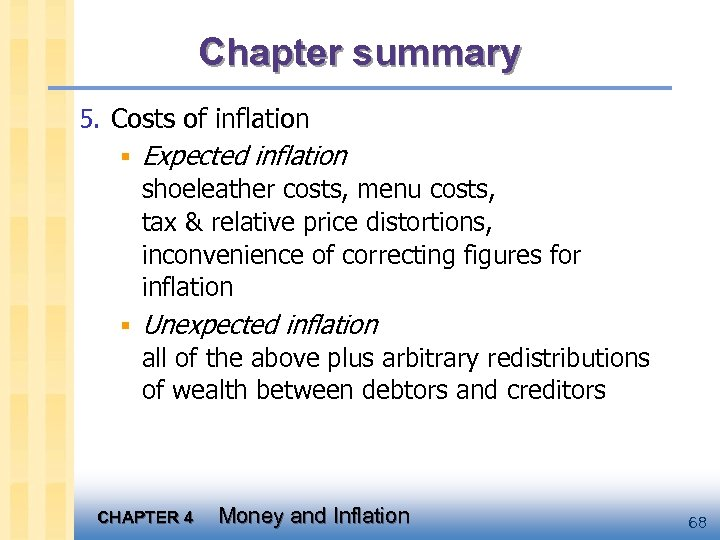Chapter summary 5. Costs of inflation § Expected inflation shoeleather costs, menu costs, tax & relative price distortions, inconvenience of correcting figures for inflation § Unexpected inflation all of the above plus arbitrary redistributions of wealth between debtors and creditors CHAPTER 4 Money and Inflation 68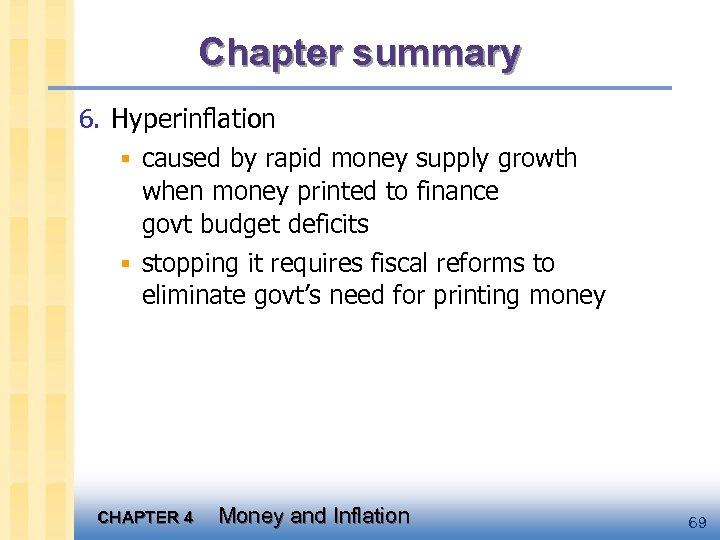Chapter summary 6. Hyperinflation § caused by rapid money supply growth when money printed to finance govt budget deficits § stopping it requires fiscal reforms to eliminate govt’s need for printing money CHAPTER 4 Money and Inflation 69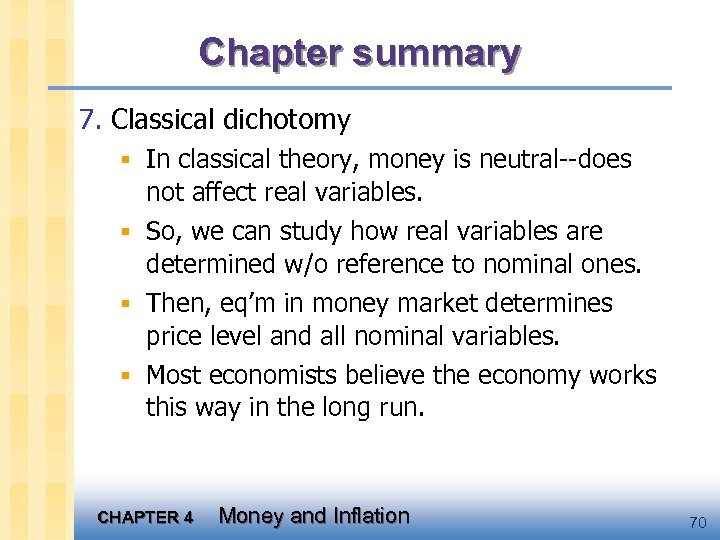Chapter summary 7. Classical dichotomy § In classical theory, money is neutral--does not affect real variables. § So, we can study how real variables are determined w/o reference to nominal ones. § Then, eq’m in money market determines price level and all nominal variables. § Most economists believe the economy works this way in the long run. CHAPTER 4 Money and Inflation 70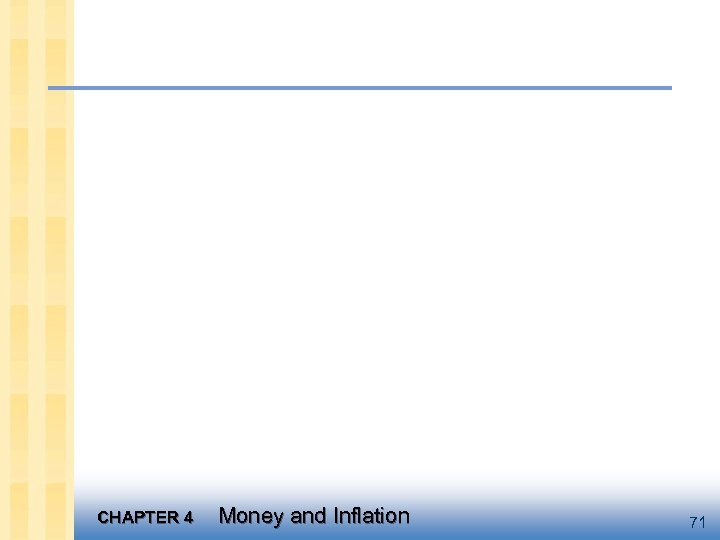CHAPTER 4 Money and Inflation 71